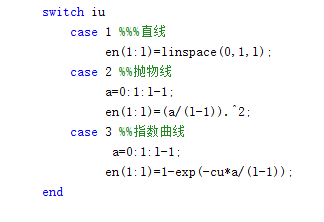matlab小白想请教一个也许各位大神看来很弱智的问题`````` switch iu
case 1 %%%直线
en(1:l)=linspace(0,1,l);
case 2 %%抛物线
a=0:1:l-1;
en(1:l)=(a/(l-1)).^2;
case 3 %%指数曲线
a=0:1:l-1;
en(1:l)=1-exp(-cu*a/(l-1));
end
``````
``````
``````

iu=fscanf(fid,'%f',1);
%iu=1; %%直线
%上升时间包络线参数(指数时候需要，其他情况均为1)

``````
``````

1个回答Bin1009 什么意思啊，请问您是说我证书过期？？这好像不是问题的关键吧。。。